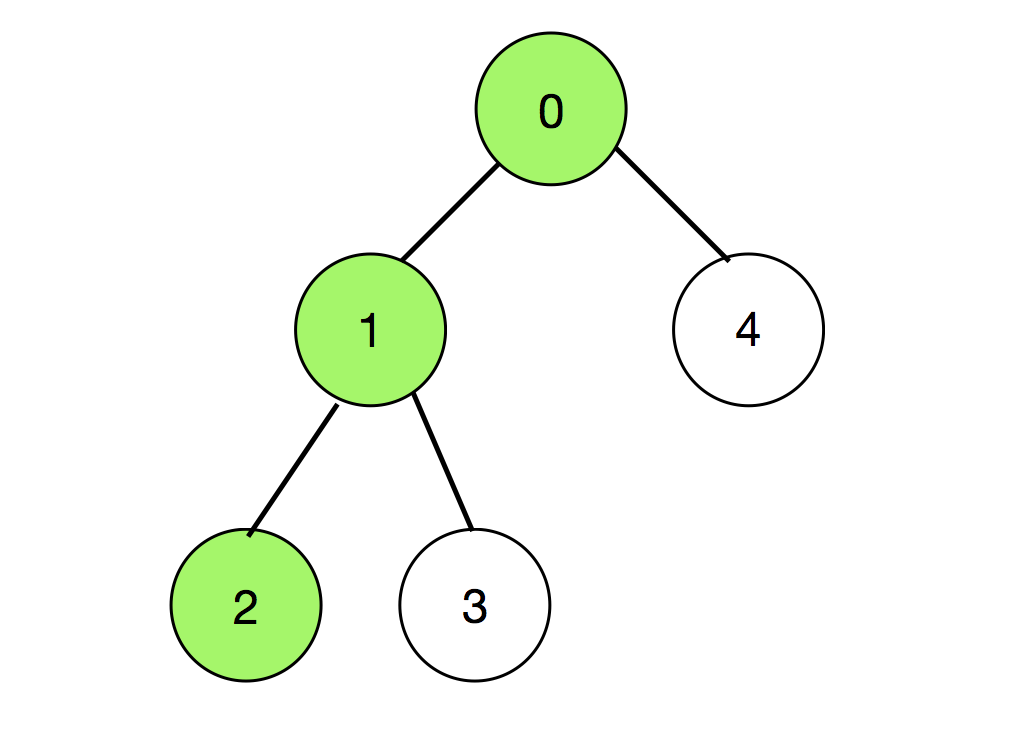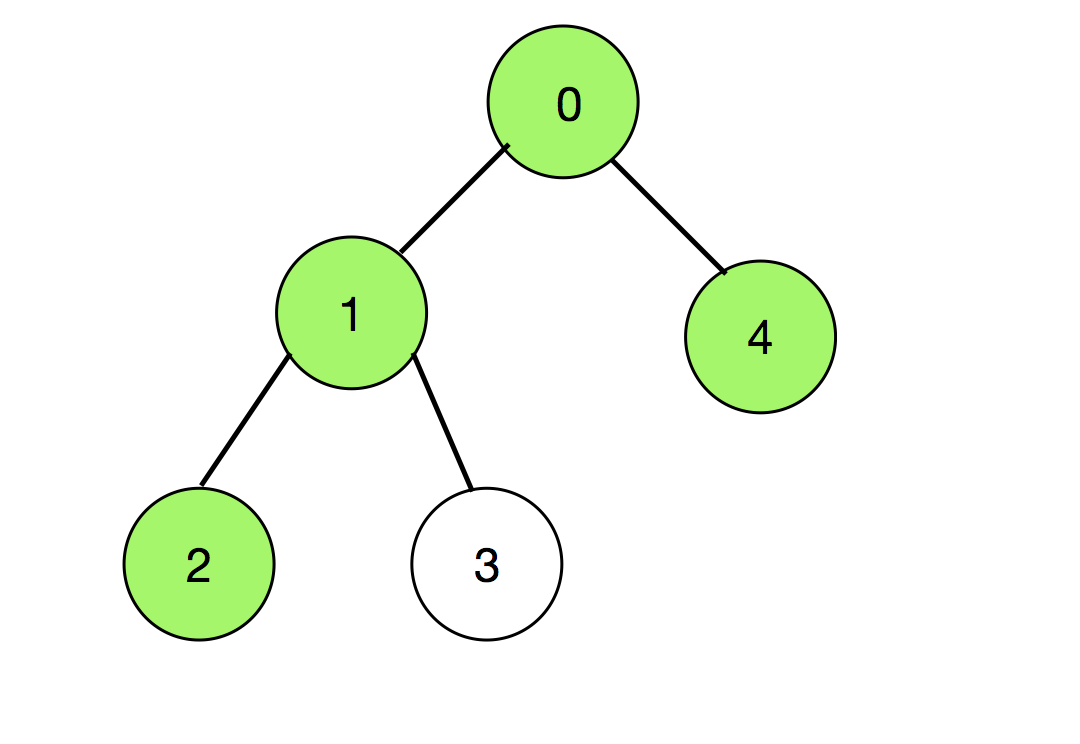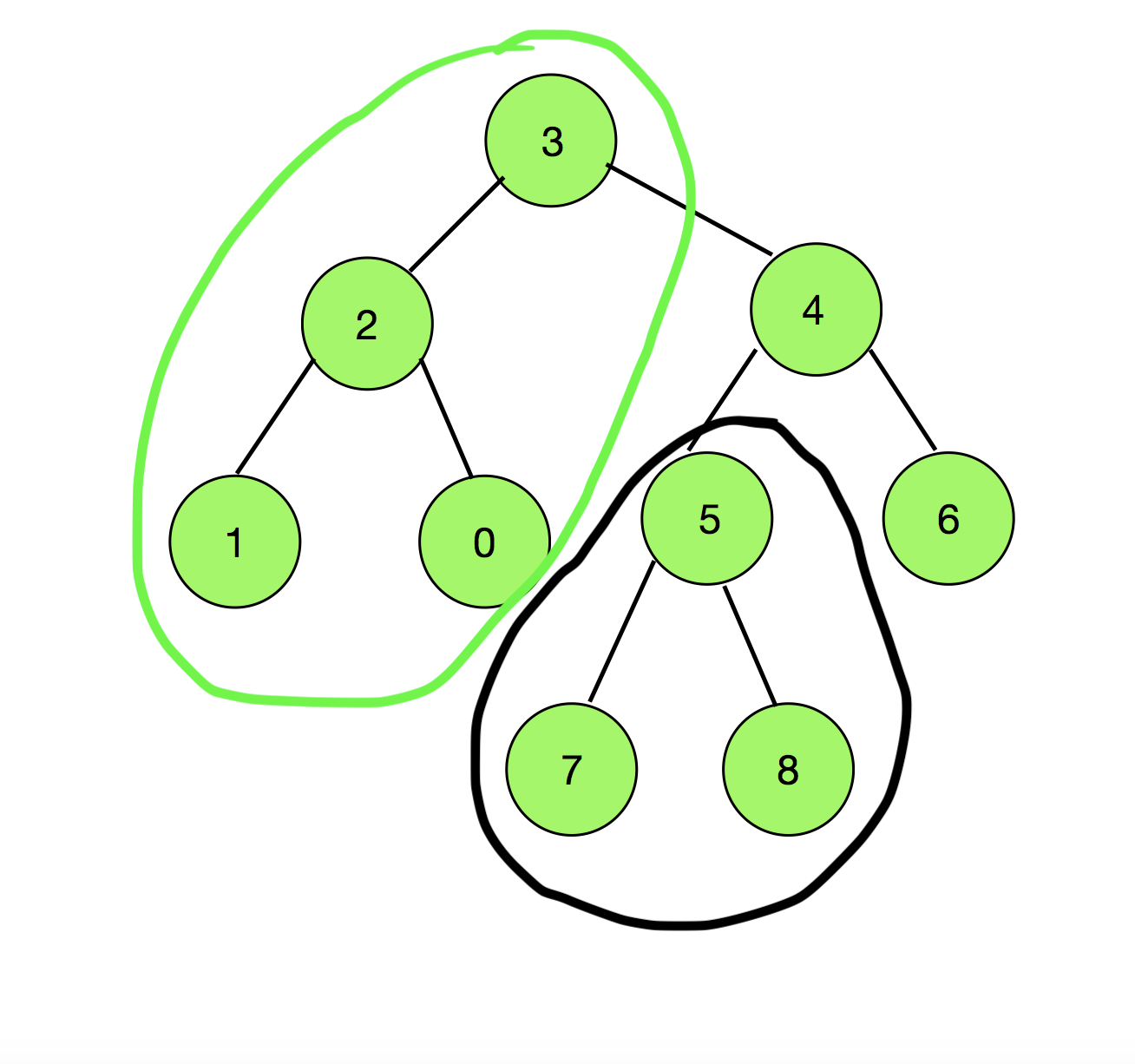# Count of all possible Paths in a Tree such that Node X does not appear before Node Y

Given a Tree consisting of N nodes having values in the range [0, N – 1] and (N – 1) edges, and two nodes X and Y, the task is to find the number of possible paths in the Tree such that the node X does not appear before the node Y in the path.

Examples:

Input: N = 5, A = 2, B = 0, Edges[][] = { {0, 1}, {1, 2}, {1, 3}, {0, 4} }
Output: 18
Explanation:
Since (X, Y) and (Y, X) are being considered different, so the count of all possible paths connecting any two pair of vertices = 2 * 5C2 = 20.
Out of these 20 pairs, those paths cannot be chosen which consist of both nodes 2 and 0 as well as Node 2 appearing before Node 0.
There are two such paths (colored as green) which are shown below:So there are total 20 – 2 = 18 such paths.
Input: N = 9, X = 5, Y = 3, Edges[][] = { {0, 2}, {1, 2}, {2, 3}, {3, 4}, {4, 6}, {4, 5}, {5, 7}, {5, 8} }
Output: 60
Explanation:
Since (X, Y) and (Y, X) are being considered different, so the count of all possible paths connecting any two pair of vertices = N * (N – 1) = 9 * 8 = 72.
On observing the diagram below, any path starting from a Node in the subtree of Node 5, denoted by black, connecting to the vertices passing through the Node 3, denoted by green, will always have 5 appearing before 3 in the path.Therefore, total number of possible paths = (Total Nodes grouped in black) * (Total Nodes grouped in green) = 3 * 4 = 12.
Therefore, the final answer = 72 – 12 = 60

Approach:
The idea is to find the combination of node pairs which will always have the Node X appearing before Node Y in the path connecting them. Then, subtract the count of such pairs from the total number of possible node pairs = NC2. Consider node Y as the root node. Now any path which first encounters X and then Y, starts from the node in the subtree of node X and ends at a node in the sub-tree of node Y but not in the subtree of node W, where W is an immediate child of node Y and lies between X and Y in these paths.

Therefore, the final answer can be calculated by:

Count = N * (N – 1) – size_of_subtree(X) * (size_of_subtree(Y) – size_of_subtree(W))

If Y is taken as the root of the tree. Then, size_of_subtree(Y) = N.

Count = N * (N – 1) – size_of_subtree(X) * (N- size_of_subtree(W))

Follow the steps below to solve the problem:

1. Initialize arrays subtree_size [], visited [] and check_subtree [] each of size N + 1. Initialize elements of visited [] as 0.
2. Perform the DFS Traversal with Y as the root node to fill the check_subtree[] and subtree_size [] for each node. The check_subtree[] checks whether the subtree of the current node contains node X or not.
3. Find the child(say node v) of Y which is in the path from X to Y. Initialize an integer variable say difference.
4. Assign (total number of nodes – subtree_size[v] ) to difference.
5. Return (N * (N – 1) ) – (subtree_size[A] * (difference)) as the answer.

Below is the implementation of the above approach:

## C++

 `// C++ Program to implement ` `// the above approach ` `#include ` `#define int long long int ` `using` `namespace` `std; ` ` `  `// Maximum number of nodes ` `const` `int` `NN = 3e5; ` ` `  `// Vector to store the tree ` `vector<``int``> G[NN + 1]; ` ` `  `// Function to perform DFS Traversal ` `int` `dfs(``int` `node, ``int` `A, ``int``* subtree_size, ` `        ``int``* visited, ``int``* check_subtree) ` `{ ` `    ``// Mark the node as visited ` `    ``visited[node] = ``true``; ` ` `  `    ``// Initialize the subtree size ` `    ``// of each node as 1 ` `    ``subtree_size[node] = 1; ` ` `  `    ``// If the node is same as A ` `    ``if` `(node == A) { ` ` `  `        ``// Mark check_subtree[node] as true ` `        ``check_subtree[node] = ``true``; ` `    ``} ` ` `  `    ``// Otherwise ` `    ``else` `        ``check_subtree[node] = ``false``; ` ` `  `    ``// Iterate over the adjacent nodes ` `    ``for` `(``int` `v : G[node]) { ` ` `  `        ``// If the adjacent node ` `        ``// is not visited ` `        ``if` `(!visited[v]) { ` ` `  `            ``// Update the size of the ` `            ``// subtree of current node ` `            ``subtree_size[node] ` `                ``+= dfs(v, A, subtree_size, ` `                       ``visited, check_subtree); ` ` `  `            ``// Check if the subtree of ` `            ``// current node contains node A ` `            ``check_subtree[node] = check_subtree[node] ` `                                  ``| check_subtree[v]; ` `        ``} ` `    ``} ` ` `  `    ``// Return size of subtree of node ` `    ``return` `subtree_size[node]; ` `} ` ` `  `// Function to add edges to the tree ` `void` `addedge(``int` `node1, ``int` `node2) ` `{ ` ` `  `    ``G[node1].push_back(node2); ` `    ``G[node2].push_back(node1); ` `} ` ` `  `// Function to calculate the number of ` `// possible paths ` `int` `numberOfPairs(``int` `N, ``int` `B, ``int` `A) ` `{ ` `    ``// Stores the size of subtree ` `    ``// of each node ` `    ``int` `subtree_size[N + 1]; ` ` `  `    ``// Stores which nodes are ` `    ``// visited ` `    ``int` `visited[N + 1]; ` ` `  `    ``// Initialise all nodes as unvisited ` `    ``memset``(visited, 0, ``sizeof``(visited)); ` ` `  `    ``// Stores if the subtree of ` `    ``// a node contains node A ` `    ``int` `check_subtree[N + 1]; ` ` `  `    ``// DFS Call ` `    ``dfs(B, A, subtree_size, ` `        ``visited, check_subtree); ` ` `  `    ``// Stores the difference between ` `    ``// total number of nodes and ` `    ``// subtree size of an immediate ` `    ``// child of Y lies between the ` `    ``// path from A to B ` `    ``int` `difference; ` ` `  `    ``// Iterate over the adjacent nodes B ` `    ``for` `(``int` `v : G[B]) { ` ` `  `        ``// If the node is in the path ` `        ``// from A to B ` `        ``if` `(check_subtree[v]) { ` ` `  `            ``// Calcualte the difference ` `            ``difference = N - subtree_size[v]; ` ` `  `            ``break``; ` `        ``} ` `    ``} ` ` `  `    ``// Return the final answer ` `    ``return` `(N * (N - 1)) ` `           ``- difference * (subtree_size[A]); ` `} ` ` `  `// Driver Code ` `int32_t main() ` `{ ` `    ``int` `N = 9; ` ` `  `    ``int` `X = 5, Y = 3; ` ` `  `    ``// Insert Edges ` `    ``addedge(0, 2); ` `    ``addedge(1, 2); ` `    ``addedge(2, 3); ` `    ``addedge(3, 4); ` `    ``addedge(4, 6); ` `    ``addedge(4, 5); ` `    ``addedge(5, 7); ` `    ``addedge(5, 8); ` ` `  `    ``cout << numberOfPairs(N, Y, X); ` ` `  `    ``return` `0; ` `} `

## Java

 `// Java Program to implement ` `// the above approach ` `import` `java.util.*; ` `class` `GFG{ ` ` `  `// Maximum number of nodes ` `static` `int` `NN = (``int``) 3e5; ` ` `  `// Vector to store the tree ` `static` `Vector []G = ``new` `Vector[NN + ``1``]; ` ` `  `// Function to perform DFS Traversal ` `static` `int` `dfs(``int` `node, ``int` `A, ``int``[] subtree_size, ` `               ``int``[] visited, ``int``[] check_subtree) ` `{ ` `    ``// Mark the node as visited ` `    ``visited[node] = ``1``; ` ` `  `    ``// Initialize the subtree size ` `    ``// of each node as 1 ` `    ``subtree_size[node] = ``1``; ` ` `  `    ``// If the node is same as A ` `    ``if` `(node == A)  ` `    ``{ ` ` `  `        ``// Mark check_subtree[node] as true ` `        ``check_subtree[node] = ``1``; ` `    ``} ` ` `  `    ``// Otherwise ` `    ``else` `        ``check_subtree[node] = ``0``; ` ` `  `    ``// Iterate over the adjacent nodes ` `    ``for` `(``int` `v : G[node]) ` `    ``{ ` ` `  `        ``// If the adjacent node ` `        ``// is not visited ` `        ``if` `(visited[v] == ``0``)  ` `        ``{ ` ` `  `            ``// Update the size of the ` `            ``// subtree of current node ` `            ``subtree_size[node] += dfs(v, A, subtree_size, ` `                                      ``visited, check_subtree); ` ` `  `            ``// Check if the subtree of ` `            ``// current node contains node A ` `            ``check_subtree[node] = check_subtree[node] |  ` `                                    ``check_subtree[v]; ` `        ``} ` `    ``} ` ` `  `    ``// Return size of subtree of node ` `    ``return` `subtree_size[node]; ` `} ` ` `  `// Function to add edges to the tree ` `static` `void` `addedge(``int` `node1, ``int` `node2) ` `{ ` `    ``G[node1].add(node2); ` `    ``G[node2].add(node1); ` `} ` ` `  `// Function to calculate the number of ` `// possible paths ` `static` `int` `numberOfPairs(``int` `N, ``int` `B, ``int` `A) ` `{ ` `    ``// Stores the size of subtree ` `    ``// of each node ` `    ``int` `[]subtree_size = ``new` `int``[N + ``1``]; ` ` `  `    ``// Stores which nodes are ` `    ``// visited ` `    ``int` `[]visited = ``new` `int``[N + ``1``]; ` ` `  ` `  `    ``// Stores if the subtree of ` `    ``// a node contains node A ` `    ``int` `[]check_subtree = ``new` `int``[N + ``1``]; ` ` `  `    ``// DFS Call ` `    ``dfs(B, A, subtree_size, ` `        ``visited, check_subtree); ` ` `  `    ``// Stores the difference between ` `    ``// total number of nodes and ` `    ``// subtree size of an immediate ` `    ``// child of Y lies between the ` `    ``// path from A to B ` `    ``int` `difference = ``0``; ` ` `  `    ``// Iterate over the adjacent nodes B ` `    ``for` `(``int` `v : G[B])  ` `    ``{ ` ` `  `        ``// If the node is in the path ` `        ``// from A to B ` `        ``if` `(check_subtree[v] > ``0``) ` `        ``{ ` ` `  `            ``// Calcualte the difference ` `            ``difference = N - subtree_size[v]; ` ` `  `            ``break``; ` `        ``} ` `    ``} ` ` `  `    ``// Return the final answer ` `    ``return` `(N * (N - ``1``)) -  ` `              ``difference * (subtree_size[A]); ` `} ` ` `  `// Driver Code ` `public` `static` `void` `main(String[] args) ` `{ ` `    ``int` `N = ``9``; ` ` `  `    ``int` `X = ``5``, Y = ``3``; ` `     `  `    ``for` `(``int` `i = ``0``; i < G.length; i++) ` `        ``G[i] = ``new` `Vector(); ` `   `  `    ``// Insert Edges ` `    ``addedge(``0``, ``2``); ` `    ``addedge(``1``, ``2``); ` `    ``addedge(``2``, ``3``); ` `    ``addedge(``3``, ``4``); ` `    ``addedge(``4``, ``6``); ` `    ``addedge(``4``, ``5``); ` `    ``addedge(``5``, ``7``); ` `    ``addedge(``5``, ``8``); ` ` `  `    ``System.out.print(numberOfPairs(N, Y, X)); ` `} ` `} ` ` `  `// This code is contributed by sapnasingh4991`

## Python3

 `# Python3 program to implement ` `# the above approach ` ` `  `# Maximum number of nodes ` `NN ``=` `int``(``3e5``) ` ` `  `# Vector to store the tree ` `G ``=` `[] ` `for` `i ``in` `range``(NN ``+` `1``): ` `    ``G.append([]) ` ` `  `# Function to perform DFS Traversal ` `def` `dfs(node, A, subtree_size,  ` `        ``visited, check_subtree): ` ` `  `    ``# Mark the node as visited ` `    ``visited[node] ``=` `True` ` `  `    ``# Initialize the subtree size ` `    ``# of each node as 1 ` `    ``subtree_size[node] ``=` `1` ` `  `    ``# If the node is same as A ` `    ``if` `(node ``=``=` `A): ` ` `  `        ``# Mark check_subtree[node] as true ` `        ``check_subtree[node] ``=` `True` ` `  `    ``# Otherwise ` `    ``else``: ` `        ``check_subtree[node] ``=` `False` ` `  `    ``# Iterate over the adjacent nodes ` `    ``for` `v ``in` `G[node]: ` ` `  `        ``# If the adjacent node ` `        ``# is not visited ` `        ``if` `(``not` `visited[v]): ` ` `  `            ``# Update the size of the ` `            ``# subtree of current node ` `            ``subtree_size[node] ``+``=` `dfs(v, A, ` `                                      ``subtree_size, ` `                                      ``visited,  ` `                                      ``check_subtree) ` ` `  `            ``# Check if the subtree of ` `            ``# current node contains node A ` `            ``check_subtree[node] ``=` `(check_subtree[node] |  ` `                                   ``check_subtree[v]) ` ` `  `    ``# Return size of subtree of node ` `    ``return` `subtree_size[node] ` ` `  `# Function to add edges to the tree ` `def` `addedge(node1, node2): ` ` `  `    ``G[node1] ``+``=` `[node2] ` `    ``G[node2] ``+``=` `[node1] ` ` `  `# Function to calculate the number of ` `# possible paths ` `def` `numberOfPairs(N, B, A): ` ` `  `    ``# Stores the size of subtree ` `    ``# of each node ` `    ``subtree_size ``=` `[``0``] ``*` `(N ``+` `1``) ` ` `  `    ``# Stores which nodes are ` `    ``# visited ` `    ``visited ``=` `[``0``] ``*` `(N ``+` `1``) ` ` `  `    ``# Stores if the subtree of ` `    ``# a node contains node A ` `    ``check_subtree ``=` `[``0``] ``*` `(N ``+` `1``) ` ` `  `    ``# DFS Call ` `    ``dfs(B, A, subtree_size, ` `        ``visited, check_subtree) ` ` `  `    ``# Stores the difference between ` `    ``# total number of nodes and ` `    ``# subtree size of an immediate ` `    ``# child of Y lies between the ` `    ``# path from A to B ` `    ``difference ``=` `0` ` `  `    ``# Iterate over the adjacent nodes B ` `    ``for` `v ``in` `G[B]: ` ` `  `        ``# If the node is in the path ` `        ``# from A to B ` `        ``if` `(check_subtree[v]): ` ` `  `            ``# Calcualte the difference ` `            ``difference ``=` `N ``-` `subtree_size[v] ` `            ``break` ` `  `    ``# Return the final answer ` `    ``return` `((N ``*` `(N ``-` `1``)) ``-`  `               ``difference ``*` `(subtree_size[A])) ` ` `  `# Driver Code ` `N ``=` `9` `X ``=` `5` `Y ``=` `3` ` `  `# Insert Edges  ` `addedge(``0``, ``2``) ` `addedge(``1``, ``2``) ` `addedge(``2``, ``3``) ` `addedge(``3``, ``4``) ` `addedge(``4``, ``6``) ` `addedge(``4``, ``5``) ` `addedge(``5``, ``7``) ` `addedge(``5``, ``8``) ` ` `  `# Function call ` `print``(numberOfPairs(N, Y, X)) ` ` `  `# This code is contributed by Shivam Singh `

## C#

 `// C# Program to implement ` `// the above approach ` `using` `System; ` `using` `System.Collections.Generic; ` ` `  `class` `GFG{ ` ` `  `// Maximum number of nodes ` `static` `int` `NN = (``int``) 3e5; ` ` `  `// List to store the tree ` `static` `List<``int``> []G = ``new` `List<``int``>[NN + 1]; ` ` `  `// Function to perform DFS Traversal ` `static` `int` `dfs(``int` `node, ``int` `A, ``int``[] subtree_size, ` `               ``int``[] visited, ``int``[] check_subtree) ` `{ ` `    ``// Mark the node as visited ` `    ``visited[node] = 1; ` ` `  `    ``// Initialize the subtree size ` `    ``// of each node as 1 ` `    ``subtree_size[node] = 1; ` ` `  `    ``// If the node is same as A ` `    ``if` `(node == A)  ` `    ``{ ` ` `  `        ``// Mark check_subtree[node] as true ` `        ``check_subtree[node] = 1; ` `    ``} ` ` `  `    ``// Otherwise ` `    ``else` `        ``check_subtree[node] = 0; ` ` `  `    ``// Iterate over the adjacent nodes ` `    ``foreach` `(``int` `v ``in` `G[node]) ` `    ``{ ` ` `  `        ``// If the adjacent node ` `        ``// is not visited ` `        ``if` `(visited[v] == 0)  ` `        ``{ ` ` `  `            ``// Update the size of the ` `            ``// subtree of current node ` `            ``subtree_size[node] += dfs(v, A, subtree_size, ` `                                      ``visited, check_subtree); ` ` `  `            ``// Check if the subtree of ` `            ``// current node contains node A ` `            ``check_subtree[node] = check_subtree[node] |  ` `                                    ``check_subtree[v]; ` `        ``} ` `    ``} ` ` `  `    ``// Return size of subtree of node ` `    ``return` `subtree_size[node]; ` `} ` ` `  `// Function to add edges to the tree ` `static` `void` `addedge(``int` `node1, ``int` `node2) ` `{ ` `    ``G[node1].Add(node2); ` `    ``G[node2].Add(node1); ` `} ` ` `  `// Function to calculate the number of ` `// possible paths ` `static` `int` `numberOfPairs(``int` `N, ``int` `B, ``int` `A) ` `{ ` `    ``// Stores the size of subtree ` `    ``// of each node ` `    ``int` `[]subtree_size = ``new` `int``[N + 1]; ` ` `  `    ``// Stores which nodes are ` `    ``// visited ` `    ``int` `[]visited = ``new` `int``[N + 1]; ` ` `  ` `  `    ``// Stores if the subtree of ` `    ``// a node contains node A ` `    ``int` `[]check_subtree = ``new` `int``[N + 1]; ` ` `  `    ``// DFS Call ` `    ``dfs(B, A, subtree_size, ` `        ``visited, check_subtree); ` ` `  `    ``// Stores the difference between ` `    ``// total number of nodes and ` `    ``// subtree size of an immediate ` `    ``// child of Y lies between the ` `    ``// path from A to B ` `    ``int` `difference = 0; ` ` `  `    ``// Iterate over the adjacent nodes B ` `    ``foreach` `(``int` `v ``in` `G[B])  ` `    ``{ ` ` `  `        ``// If the node is in the path ` `        ``// from A to B ` `        ``if` `(check_subtree[v] > 0) ` `        ``{ ` ` `  `            ``// Calcualte the difference ` `            ``difference = N - subtree_size[v]; ` ` `  `            ``break``; ` `        ``} ` `    ``} ` ` `  `    ``// Return the readonly answer ` `    ``return` `(N * (N - 1)) -  ` `              ``difference * (subtree_size[A]); ` `} ` ` `  `// Driver Code ` `public` `static` `void` `Main(String[] args) ` `{ ` `    ``int` `N = 9; ` ` `  `    ``int` `X = 5, Y = 3; ` `     `  `    ``for` `(``int` `i = 0; i < G.Length; i++) ` `        ``G[i] = ``new` `List<``int``>(); ` `   `  `    ``// Insert Edges ` `    ``addedge(0, 2); ` `    ``addedge(1, 2); ` `    ``addedge(2, 3); ` `    ``addedge(3, 4); ` `    ``addedge(4, 6); ` `    ``addedge(4, 5); ` `    ``addedge(5, 7); ` `    ``addedge(5, 8); ` ` `  `    ``Console.Write(numberOfPairs(N, Y, X)); ` `} ` `} ` ` `  ` `  `// This code is contributed by sapnasingh4991`

Output:

```60

```

Time Complexity: O(N)
Auxiliary Space: O(N)

Attention reader! Don’t stop learning now. Get hold of all the important DSA concepts with the DSA Self Paced Course at a student-friendly price and become industry ready.

My Personal Notes arrow_drop_upCheck out this Author's contributed articles.

If you like GeeksforGeeks and would like to contribute, you can also write an article using contribute.geeksforgeeks.org or mail your article to contribute@geeksforgeeks.org. See your article appearing on the GeeksforGeeks main page and help other Geeks.

Please Improve this article if you find anything incorrect by clicking on the "Improve Article" button below.